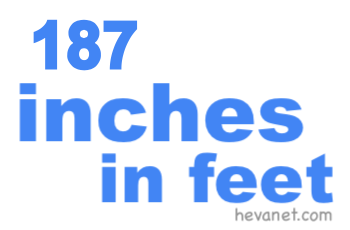187 inches in feetHere we will show you how to get 187 inches in feet (187 in to ft). We will show you how to convert 187 inches in feet as a decimal, 187 inches in feet and inches as a decimal, 187 inches in feet as a fraction, and finally, 187 inches in feet and inches as a fraction.

187 inches in feet as a decimal
We start with the easiest conversion. There are twelve inches per foot. Thus, to convert 187 inches to feet as a decimal, you can simply divide 187 by 12 like this:

187 ÷ 12 ≈ 15.5833333333333
187 inches ≈ 15.583 feet

187 inches in feet and inches as a decimal
187 inches makes 15 full feet per our calculation above. We subtract the full feet from 187 to get the remaining inches. Below is the math and the answer.

187 - (15 × 12) = 7
187 inches = 15 feet and 7 inches

187 inches in feet as a fraction
You already have the whole number of feet from the answer above. To get the fractional number in feet, you put the decimal inches number from the answer above over 12 and then simplify:

7/12 = 70/120 = 7/12
187 inches = 15 7/12 feet

187 inches in feet and inches as a fraction
Again, you already have the whole number of feet from the answer above. To get the fractional inches, you put the decimal inches number from the answer above over 1 and then simplify:

7/1 = 70/10 = 7
187 inches = 15 feet and 7 inches

Inches in Feet
Enter another length of inches below to see what it is in feet.

187.1 inches in feet
Go here for the next length in inches on our list that we have converted to feet for you.